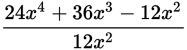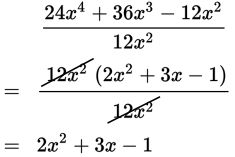# SAT Math Multiple Choice Question 242: Answer and Explanation

### Test Information

Question: 242

2.Which of the following expressions is equivalent to the expression shown above?

• A. 2x2 + 3x
• B. 24x4 + 36x3
• C. 2x2 + 3x - 1
• D. 24x4 + 36x3 - 1

Explanation:

C

Difficulty: Easy

Category: Passport to Advanced Math / Exponents

Strategic Advice: Don't be tempted-you can't simply cancel one term when a polynomial is divided by a monomial. Instead, find the greatest common factor of both the numerator and the denominator. Factor out the GCF from the numerator and from the denominator, and then you can cancel it.

Getting to the Answer: The GCF is 12x2.